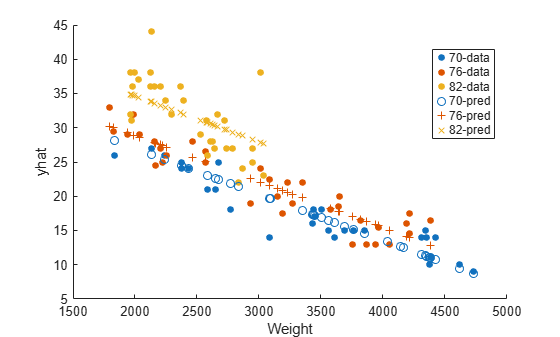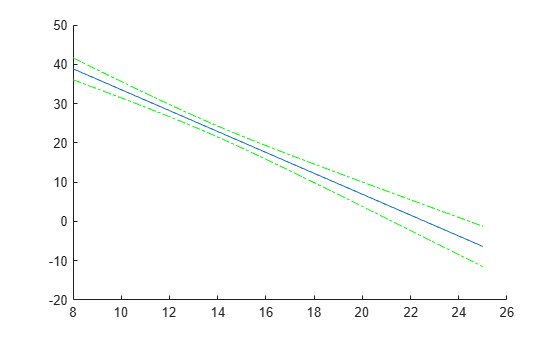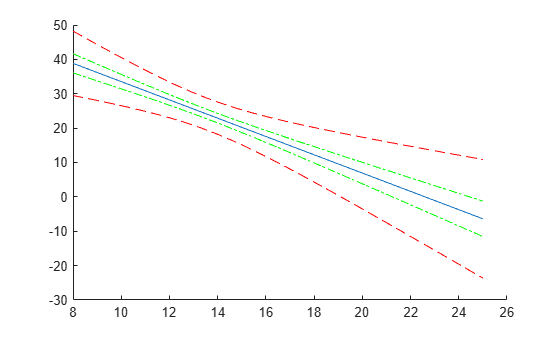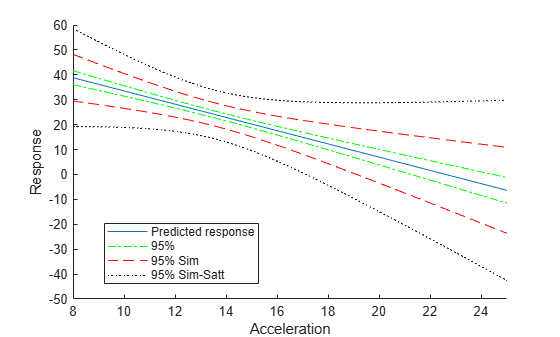Main Content

# predict

Class: LinearMixedModel

Predict response of linear mixed-effects model

## Syntax

``ypred = predict(lme)``
``ypred = predict(lme,tblnew)``
``ypred = predict(lme,Xnew,Znew)``
``ypred = predict(lme,Xnew,Znew,Gnew)``
``ypred = predict(___,Name,Value)``
``````[ypred,ypredCI] = predict(___)``````
``````[ypred,ypredCI,DF] = predict(___)``````

## Description

example

````ypred = predict(lme)` returns a vector of conditional predicted responses `ypred` at the original predictors used to fit the linear mixed-effects model `lme`.```

example

````ypred = predict(lme,tblnew)` returns a vector of conditional predicted responses `ypred` from the fitted linear mixed-effects model `lme` at the values in the new table or dataset array `tblnew`. Use a table or dataset array for `predict` if you use a table or dataset array for fitting the model `lme`.If a particular grouping variable in `tblnew` has levels that are not in the original data, then the random effects for that grouping variable do not contribute to the `'Conditional'` prediction at observations where the grouping variable has new levels.```
````ypred = predict(lme,Xnew,Znew)` returns a vector of conditional predicted responses `ypred` from the fitted linear mixed-effects model `lme` at the values in the new fixed- and random-effects design matrices, `Xnew` and `Znew`, respectively. `Znew` can also be a cell array of matrices. In this case, the grouping variable `G` is `ones(n,1)`, where n is the number of observations used in the fit. Use the matrix format for `predict` if using design matrices for fitting the model `lme`.```

example

````ypred = predict(lme,Xnew,Znew,Gnew)` returns a vector of conditional predicted responses `ypred` from the fitted linear mixed-effects model `lme` at the values in the new fixed- and random-effects design matrices, `Xnew` and `Znew`, respectively, and the grouping variable `Gnew`.`Znew` and `Gnew` can also be cell arrays of matrices and grouping variables, respectively.```

example

````ypred = predict(___,Name,Value)` returns a vector of predicted responses `ypred` from the fitted linear mixed-effects model `lme` with additional options specified by one or more `Name,Value` pair arguments.For example, you can specify the confidence level, simultaneous confidence bounds, or contributions from only fixed effects.```

example

``````[ypred,ypredCI] = predict(___)``` also returns confidence intervals `ypredCI` for the predictions `ypred` for any of the input arguments in the previous syntaxes.```

example

``````[ypred,ypredCI,DF] = predict(___)``` also returns the degrees of freedom `DF` used in computing the confidence intervals for any of the input arguments in the previous syntaxes.```

## Input Arguments

expand all

Linear mixed-effects model, specified as a `LinearMixedModel` object constructed using `fitlme` or `fitlmematrix`.

New input data, which includes the response variable, predictor variables, and grouping variables, specified as a table or dataset array. The predictor variables can be continuous or grouping variables. `tblnew` must have the same variables as in the original table or dataset array used to fit the linear mixed-effects model `lme`.

New fixed-effects design matrix, specified as an n-by-p matrix, where n is the number of observations and p is the number of fixed predictor variables. Each row of `X` corresponds to one observation and each column of `X` corresponds to one variable.

Data Types: `single` | `double`

New random-effects design, specified as an n-by-q matrix or a cell array of R design matrices `Z{r}`, where r = 1, 2, ..., R. If `Znew` is a cell array, then each `Z{r}` is an n-by-q(r) matrix, where n is the number of observations, and q(r) is the number of random predictor variables.

Data Types: `single` | `double` | `cell`

New grouping variable or variables, specified as a vector or a cell array, of length R, of grouping variables with the same levels or groups as the original grouping variables used to fit the linear mixed-effects model `lme`.

Data Types: `single` | `double` | `categorical` | `logical` | `char` | `string` | `cell`

### Name-Value Pair Arguments

Specify optional comma-separated pairs of `Name,Value` arguments. `Name` is the argument name and `Value` is the corresponding value. `Name` must appear inside quotes. You can specify several name and value pair arguments in any order as `Name1,Value1,...,NameN,ValueN`.

Significance level, specified as the comma-separated pair consisting of `'Alpha'` and a scalar value in the range 0 to 1. For a value α, the confidence level is 100*(1–α)%.

For example, for 99% confidence intervals, you can specify the confidence level as follows.

Example: `'Alpha',0.01`

Data Types: `single` | `double`

Indicator for conditional predictions, specified as the comma-separated pair consisting of `'Conditional'` and one of the following.

 `true` Contributions from both fixed effects and random effects (conditional) `false` Contribution from only fixed effects (marginal)

Example: `'Conditional,false`

Method for computing approximate degrees of freedom to use in the confidence interval computation, specified as the comma-separated pair consisting of `'DFMethod'` and one of the following.

 `'residual'` Default. The degrees of freedom are assumed to be constant and equal to n – p, where n is the number of observations and p is the number of fixed effects. `'satterthwaite'` Satterthwaite approximation. `'none'` All degrees of freedom are set to infinity.

For example, you can specify the Satterthwaite approximation as follows.

Example: `'DFMethod','satterthwaite'`

Type of confidence bounds, specified as the comma-separated pair consisting of `'Simultaneous'` and one of the following.

 `false` Default. Nonsimultaneous bounds. `true` Simultaneous bounds.

Example: `'Simultaneous',true`

Type of prediction, specified as the comma-separated pair consisting of `'Prediction'` and one of the following.

 `'curve'` Default. Confidence bounds for the predictions based on the fitted function. `'observation'` Variability due to observation error for the new observations is also included in the confidence bound calculations and this results in wider bounds.

Example: `'Prediction','observation'`

## Output Arguments

expand all

Predicted responses, returned as a vector. `ypred` can contain the conditional or marginal responses, depending on the value choice of the `'Conditional'` name-value pair argument. Conditional predictions include contributions from both fixed and random effects.

Point-wise confidence intervals for the predicted values, returned as a two-column matrix. The first column of `yCI` contains the lower bounds, and the second column contains the upper bound. By default, `yCI` contains the 95% confidence intervals for the predictions. You can change the confidence level using the `Alpha` name-value pair argument, make them simultaneous using the `Simultaneous` name-value pair argument, and also make them for a new observation rather than for the curve using the `Prediction` name-value pair argument.

Degrees of freedom used in computing the confidence intervals, returned as a vector or a scalar value.

• If the `'Simultaneous'` name-value pair argument is `false`, then `DF` is a vector.

• If the `'Simultaneous'` name-value pair argument is `true`, then `DF` is a scalar value.

## Examples

expand all

Load the sample data.

`load('fertilizer.mat');`

The dataset array includes data from a split-plot experiment, where soil is divided into three blocks based on the soil type: sandy, silty, and loamy. Each block is divided into five plots, where five different types of tomato plants (cherry, heirloom, grape, vine, and plum) are randomly assigned to these plots. The tomato plants in the plots are then divided into subplots, where each subplot is treated by one of four fertilizers. This is simulated data.

Store the data in a dataset array called `ds`, for practical purposes, and define `Tomato`, `Soil`, and `Fertilizer` as categorical variables.

```ds = fertilizer; ds.Tomato = nominal(ds.Tomato); ds.Soil = nominal(ds.Soil); ds.Fertilizer = nominal(ds.Fertilizer);```

Fit a linear mixed-effects model, where `Fertilizer` and `Tomato` are the fixed-effects variables, and the mean yield varies by the block (soil type), and the plots within blocks (tomato types within soil types) independently.

`lme = fitlme(ds,'Yield ~ Fertilizer * Tomato + (1|Soil) + (1|Soil:Tomato)');`

Predict the response values at the original design values. Display the first five predictions with the observed response values.

```yhat = predict(lme); [yhat(1:5) ds.Yield(1:5)]```
```ans = 5×2 115.4788 104.0000 135.1455 136.0000 152.8121 158.0000 160.4788 174.0000 58.0839 57.0000 ```

Load the sample data.

`load carsmall`

Fit a linear mixed-effects model, with a fixed effect for `Weight`, and a random intercept grouped by `Model_Year`. First, store the data in a table.

```tbl = table(MPG,Weight,Model_Year); lme = fitlme(tbl,'MPG ~ Weight + (1|Model_Year)');```

Create predicted responses to the data.

`yhat = predict(lme,tbl);`

Plot the original responses and the predicted responses to see how they differ. Group them by model year.

```figure() gscatter(Weight,MPG,Model_Year) hold on gscatter(Weight,yhat,Model_Year,[],'o+x') legend('70-data','76-data','82-data','70-pred','76-pred','82-pred') hold off```Load the sample data.

`load('fertilizer.mat');`

The dataset array includes data from a split-plot experiment, where soil is divided into three blocks based on the soil type: sandy, silty, and loamy. Each block is divided into five plots, where five different types of tomato plants (cherry, heirloom, grape, vine, and plum) are randomly assigned to these plots. The tomato plants in the plots are then divided into subplots, where each subplot is treated by one of four fertilizers. This is simulated data.

Store the data in a dataset array called `ds`, for practical purposes, and define `Tomato`, `Soil`, and `Fertilizer` as categorical variables.

```ds = fertilizer; ds.Tomato = nominal(ds.Tomato); ds.Soil = nominal(ds.Soil); ds.Fertilizer = nominal(ds.Fertilizer);```

Fit a linear mixed-effects model, where `Fertilizer` and `Tomato` are the fixed-effects variables, and the mean yield varies by the block (soil type), and the plots within blocks (tomato types within soil types) independently.

`lme = fitlme(ds,'Yield ~ Fertilizer * Tomato + (1|Soil) + (1|Soil:Tomato)');`

Create a new dataset array with design values. The new dataset array must have the same variables as the original dataset array you use for fitting the model `lme`.

```dsnew = dataset(); dsnew.Soil = nominal({'Sandy';'Silty'}); dsnew.Tomato = nominal({'Cherry';'Vine'}); dsnew. Fertilizer = nominal([2;2]);```

Predict the conditional and marginal responses at the original design points.

```yhatC = predict(lme,dsnew); yhatM = predict(lme,dsnew,'Conditional',false); [yhatC yhatM]```
```ans = 2×2 92.7505 111.6667 87.5891 82.6667 ```

Load the sample data.

`load carbig`

Fit a linear mixed-effects model for miles per gallon (MPG), with fixed effects for acceleration, horsepower, and cylinders, and potentially correlated random effects for intercept and acceleration grouped by model year.

First, prepare the design matrices for fitting the linear mixed-effects model.

```X = [ones(406,1) Acceleration Horsepower]; Z = [ones(406,1) Acceleration]; Model_Year = nominal(Model_Year); G = Model_Year;```

Now, fit the model using `fitlmematrix` with the defined design matrices and grouping variables.

```lme = fitlmematrix(X,MPG,Z,G,'FixedEffectPredictors',.... {'Intercept','Acceleration','Horsepower'},'RandomEffectPredictors',... {{'Intercept','Acceleration'}},'RandomEffectGroups',{'Model_Year'});```

Create the design matrices that contain the data at which to predict the response values. `Xnew` must have three columns as in `X`. The first column must be a column of 1s. And the values in the last two columns must correspond to `Acceleration` and `Horsepower`, respectively. The first column of `Znew` must be a column of 1s, and the second column must contain the same `Acceleration` values as in `Xnew`. The original grouping variable in `G` is the model year. So, `Gnew` must contain values for the model year. Note that `Gnew` must contain nominal values.

```Xnew = [1,13.5,185; 1,17,205; 1,21.2,193]; Znew = [1,13.5; 1,17; 1,21.2]; % alternatively Znew = Xnew(:,1:2); Gnew = nominal([73 77 82]);```

Predict the responses for the data in the new design matrices.

`yhat = predict(lme,Xnew,Znew,Gnew)`
```yhat = 3×1 8.7063 5.4423 12.5384 ```

Now, repeat the same for a linear mixed-effects model with uncorrelated random-effects terms for intercept and acceleration. First, change the original random effects design and the random effects grouping variables. Then, refit the model.

```Z = {ones(406,1),Acceleration}; G = {Model_Year,Model_Year}; lme = fitlmematrix(X,MPG,Z,G,'FixedEffectPredictors',.... {'Intercept','Acceleration','Horsepower'},'RandomEffectPredictors',... {{'Intercept'},{'Acceleration'}},'RandomEffectGroups',{'Model_Year','Model_Year'});```

Now, recreate the new random effects design, `Znew`, and the grouping variable design, `Gnew`, using which to predict the response values.

```Znew = {[1;1;1],[13.5;17;21.2]}; MY = nominal([73 77 82]); Gnew = {MY,MY};```

Predict the responses using the new design matrices.

`yhat = predict(lme,Xnew,Znew,Gnew)`
```yhat = 3×1 8.6365 5.9199 12.1247 ```

Load the sample data.

`load carbig`

Fit a linear mixed-effects model for miles per gallon (MPG), with fixed effects for acceleration, horsepower, and cylinders, and potentially correlated random effects for intercept and acceleration grouped by model year. First, store the variables in a table.

`tbl = table(MPG,Acceleration,Horsepower,Model_Year);`

Now, fit the model using `fitlme` with the defined design matrices and grouping variables.

`lme = fitlme(tbl,'MPG ~ Acceleration + Horsepower + (Acceleration|Model_Year)');`

Create the new data and store it in a new table.

```tblnew = table(); tblnew.Acceleration = linspace(8,25)'; tblnew.Horsepower = linspace(min(Horsepower),max(Horsepower))'; tblnew.Model_Year = repmat(70,100,1);```

`linspace` creates 100 equally distanced values between the lower and the upper input limits. `Model_Year` is fixed at 70. You can repeat this for any model year.

Compute and plot the predicted values and 95% confidence limits (nonsimultaneous).

```[ypred,yCI,DF] = predict(lme,tblnew); figure(); h1 = line(tblnew.Acceleration,ypred); hold on; h2 = plot(tblnew.Acceleration,yCI,'g-.');```Display the degrees of freedom.

`DF(1)`
```ans = 389 ```

Compute and plot the simultaneous confidence bounds.

```[ypred,yCI,DF] = predict(lme,tblnew,'Simultaneous',true); h3 = plot(tblnew.Acceleration,yCI,'r--');```Display the degrees of freedom.

`DF`
```DF = 389 ```

Compute the simultaneous confidence bounds using the Satterthwaite method to compute the degrees of freedom.

```[ypred,yCI,DF] = predict(lme,tblnew,'Simultaneous',true,'DFMethod','satterthwaite'); h4 = plot(tblnew.Acceleration,yCI,'k:'); hold off xlabel('Acceleration') ylabel('Response') ylim([-50,60]) xlim([8,25]) legend([h1,h2(1),h3(1),h4(1)],'Predicted response','95%','95% Sim',... '95% Sim-Satt','Location','Best')```Display the degrees of freedom.

`DF`
```DF = 3.6001 ```

expand all

Download ebook